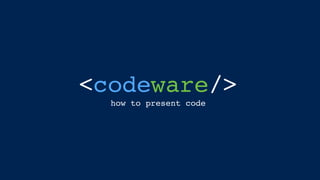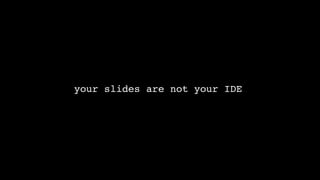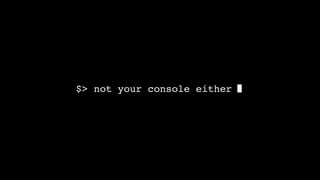Successfully reported this slideshow.

# Codeware

199

Share×
1 of 67
1 of 67

# Codeware

199

Share

How to present code.

How to present code.

## More Related Content

### Related Books

Free with a 14 day trial from Scribd

See all

### Related Audiobooks

Free with a 14 day trial from Scribd

See all

### Codeware

1. 1. <codeware/> how to present code
3. 3. \$> not your console either
4. 4. here are five rules for presenting code
5. 5. rule 1: \$ use monospaced font \$
6. 6. or monospace proportional
7. 7. monospace proportional fixed or variable ﬁxed or variable
8. 8. var power = function(base, exponent) { var result = 1; for (var count = 0; count < exponent; count++) result *= base; return result; }; var power = function(base, exponent) { var result = 1; for (var count = 0; count < exponent; count++) result *= base; return result; }; monospace proportional
9. 9. var power = function(base, exponent) { var result = 1; for (var count = 0; count < exponent; count++) result *= base; return result; }; var power = function(base, exponent) { var result = 1; for (var count = 0; count < exponent; count++) result *= base; return result; }; monospace proportional
10. 10. monospace monospaced fonts have better readability when presenting code proportional
11. 11. monospace proportional it is harder to follow code with proportional fonts monospaced fonts have better readability when presenting code
12. 12. use monospaced fonts for code readability
13. 13. rule 2: \$ use big fonts \$
14. 14. presenting for a big audience? \$ this is not your desktop monitor \$ font size should be bigger \$
15. 15. I mean BIGGER than your think
16. 16. I really mean BIGGER  than your think
17. 17. console.log(“even BIGGER where possible”)
18. 18. console .log(“use line breaks”)
19. 19. <body> <button onClick=“coolFunction()” autofocus>too small</button> </body>
20. 20. <body> <button onClick=“coolFunction()” autofocus> same but BIGGER </button> </body>
21. 21. BIGGER is betterhow do you expect people in the back to read this?
22. 22. rule 3: \$ smart syntax  highlighting \$
23. 23. this is not your IDE use syntax highlighting only where needed
24. 24. this is not your IDE use syntax highlighting only where needed
25. 25. Presenting code on your slides usually results in a wall of text. Highlight only the  specific parts you want your audience to focus on.
26. 26. Presenting code on your slides usually results in a wall of text. Highlight only the  specific parts you want your audience to focus on.
27. 27. Presenting code on your slides usually results in a wall of text. Highlight only the  specific parts you want your audience to focus on.
28. 28. 3 slides example - Ruby map function 1 2 3
29. 29. names = ['rey', 'fin', 'poe'] names.map! {|name| name.capitalize } puts names 3 slides example - Ruby map function 1 2 3
30. 30. names = ['rey', 'fin', 'poe'] names.map! {|name| name.capitalize } puts names 3 slides example - Ruby map function 1 2 3
31. 31. names = ['rey', 'fin', 'poe'] names.map! {|name| name.capitalize } puts names # output Rey Fin Poe 3 slides example - Ruby map function 1 2 3
32. 32. rule 4: \$ using ellipsis… \$
33. 33. import sys from ortools.constraint_solver import pywrapcp # By default, solve the 8x8 problem. n = 8 if len(sys.argv) < 2 else int(sys.argv) # Creates the solver. solver = pywrapcp.Solver("n-queens") # Creates the variables. # The array index is the row, and the value is the column. queens = [solver.IntVar(0, n - 1, "x%i" % i) for i in range(n)] # Creates the constraints. # All columns must be different. # (The "not on the same row" constraint is implicit from the array we're # using to store our variables; since no two elements of an array # can have the same index, there can be no queens with the same row.) solver.Add(solver.AllDifferent(queens)) # No two queens can be on the same diagonal. solver.Add(solver.AllDifferent([queens[i] + i for i in range(n)])) solver.Add(solver.AllDifferent([queens[i] - i for i in range(n)])) db = solver.Phase(queens, solver.CHOOSE_MIN_SIZE_LOWEST_MAX, solver.ASSIGN_CENTER_VALUE) solver.NewSearch(db) # Iterates through the solutions, displaying each. num_solutions = 0 while solver.NextSolution(): queen_columns = [int(queens[i].Value()) for i in range(n)] # Displays the solution just computed. for i in range(n): for j in range(n): if queen_columns[i] == j: print "Q", else: print "_", print print num_solutions += 1 solver.EndSearch() print print "Solutions found:", num_solutions print "Time:", solver.WallTime(), "ms" Solving the N-queens Problem
34. 34. import sys from ortools.constraint_solver import pywrapcp # By default, solve the 8x8 problem. n = 8 if len(sys.argv) < 2 else int(sys.argv) # Creates the solver. solver = pywrapcp.Solver("n-queens") # Creates the variables. # The array index is the row, and the value is the column. queens = [solver.IntVar(0, n - 1, "x%i" % i) for i in range(n)] # Creates the constraints. # All columns must be different. # (The "not on the same row" constraint is implicit from the array we're # using to store our variables; since no two elements of an array # can have the same index, there can be no queens with the same row.) solver.Add(solver.AllDifferent(queens)) # No two queens can be on the same diagonal. solver.Add(solver.AllDifferent([queens[i] + i for i in range(n)])) solver.Add(solver.AllDifferent([queens[i] - i for i in range(n)])) db = solver.Phase(queens, solver.CHOOSE_MIN_SIZE_LOWEST_MAX, solver.ASSIGN_CENTER_VALUE) solver.NewSearch(db) # Iterates through the solutions, displaying each. num_solutions = 0 while solver.NextSolution(): queen_columns = [int(queens[i].Value()) for i in range(n)] # Displays the solution just computed. for i in range(n): for j in range(n): if queen_columns[i] == j: print "Q", else: print "_", print print num_solutions += 1 solver.EndSearch() print print "Solutions found:", num_solutions print "Time:", solver.WallTime(), "ms" Solving the N-queens Problem what’s the point in presenting all you code if people can’t follow?
35. 35. import sys from ortools.constraint_solver import pywrapcp # By default, solve the 8x8 problem. n = 8 if len(sys.argv) < 2 else int(sys.argv) # Creates the solver. solver = pywrapcp.Solver("n-queens") # Creates the variables. # The array index is the row, and the value is the column. queens = [solver.IntVar(0, n - 1, "x%i" % i) for i in range(n)] # Creates the constraints. # All columns must be different. # (The "not on the same row" constraint is implicit from the array we're # using to store our variables; since no two elements of an array # can have the same index, there can be no queens with the same row.) solver.Add(solver.AllDifferent(queens)) # No two queens can be on the same diagonal. solver.Add(solver.AllDifferent([queens[i] + i for i in range(n)])) solver.Add(solver.AllDifferent([queens[i] - i for i in range(n)])) db = solver.Phase(queens, solver.CHOOSE_MIN_SIZE_LOWEST_MAX, solver.ASSIGN_CENTER_VALUE) solver.NewSearch(db) # Iterates through the solutions, displaying each. num_solutions = 0 while solver.NextSolution(): queen_columns = [int(queens[i].Value()) for i in range(n)] # Displays the solution just computed. for i in range(n): for j in range(n): if queen_columns[i] == j: print "Q", else: print "_", print print num_solutions += 1 solver.EndSearch() print print "Solutions found:", num_solutions print "Time:", solver.WallTime(), "ms" Solving the N-queens Problem keep just the relevant code
36. 36. import sys from ortools.constraint_solver import pywrapcp # By default, solve the 8x8 problem. n = 8 if len(sys.argv) < 2 else int(sys.argv) # Creates the solver. solver = pywrapcp.Solver("n-queens") # Creates the variables. # The array index is the row, and the value is the column. queens = [solver.IntVar(0, n - 1, "x%i" % i) for i in range(n)] # Creates the constraints. # All columns must be different. # (The "not on the same row" constraint is implicit from the array we're # using to store our variables; since no two elements of an array # can have the same index, there can be no queens with the same row.) solver.Add(solver.AllDifferent(queens)) # No two queens can be on the same diagonal. solver.Add(solver.AllDifferent([queens[i] + i for i in range(n)])) solver.Add(solver.AllDifferent([queens[i] - i for i in range(n)])) db = solver.Phase(queens, solver.CHOOSE_MIN_SIZE_LOWEST_MAX, solver.ASSIGN_CENTER_VALUE) solver.NewSearch(db) # Iterates through the solutions, displaying each. num_solutions = 0 while solver.NextSolution(): queen_columns = [int(queens[i].Value()) for i in range(n)] # Displays the solution just computed. for i in range(n): for j in range(n): if queen_columns[i] == j: print "Q", else: print "_", print print num_solutions += 1 solver.EndSearch() print print "Solutions found:", num_solutions print "Time:", solver.WallTime(), "ms" Solving the N-queens Problem use ellipsis…
37. 37. ... # Creates the solver. solver = pywrapcp.Solver("n-queens") ... # Iterates through the solutions, displaying each. num_solutions = 0 while solver.NextSolution(): queen_columns = [int(queens[i].Value()) for i in range(n)] # Displays the solution just computed. for i in range(n): for j in range(n): if queen_columns[i] == j: print "Q", else: print "_", print print num_solutions += 1 solver.EndSearch() ... Solving the N-queens Problem
38. 38. ... # Creates the solver. solver = pywrapcp.Solver("n-queens") ... # Iterates through the solutions, displaying each. num_solutions = 0 while solver.NextSolution(): queen_columns = [int(queens[i].Value()) for i in range(n)] # Displays the solution just computed. for i in range(n): for j in range(n): if queen_columns[i] == j: print "Q", else: print "_", print print num_solutions += 1 solver.EndSearch() ... Solving the N-queens Problem the focus is now on the algorithm  not the boilerplate
39. 39. rule 5: \$ use screen annotation \$
40. 40. do you want to focus your audience’s attention on a specific area in the screen?
41. 41. thinking of using a laser pointer?
42. 42. do you expect your audience to track this?
43. 43. always create slides as if you are the person sitting  in the last row of the conference hall. use screen annotations.
44. 44. always create slides as if you are the person sitting  in the last row of the conference hall. use screen annotations.
45. 45. always create slides as if you are the person sitting  in the last row of the conference hall. use screen annotations.
46. 46. always create slides as if you are the person sitting  in the last row of the conference hall. use screen annotations.
47. 47. putting it all together \$ ECMAScript 2015 Promise example \$
48. 48. putting it all together \$ ECMAScript 2015 Promise example \$ the next slides will introduce the  ECMAScript 2015 Promise Object
49. 49. fetchGraphData(graphName, function(error, graphData)) { if (error) { // handle error } // ... renderGraph(graphData, function(error)) { if (error) { // handle error } // ... notifyClients(msg, result, function(error, response)) { if (error) { // handle error } // ... }); }); }); Typical JS code results with  many nested callbacks
50. 50. fetchGraphData(graphName, function(error, graphData)) { if (error) { // handle error } // ... renderGraph(graphData, function(error)) { if (error) { // handle error } // ... notifyClients(msg, result, function(error, response)) { if (error) { // handle error } // ... }); }); }); makes it hard to follow
51. 51. fetchGraphData(graphName, function(error, graphData)) { if (error) { // handle error } // ... renderGraph(graphData, function(error)) { if (error) { // handle error } // ... notifyClients(msg, result, function(error, response)) { if (error) { // handle error } // ... }); }); }); error handling is duplicated
52. 52. ECMAScript 2015 Promise to the rescue!
53. 53. function fetchGraphData(graphName) { return new Promise(function(resolve, reject)) { let request = new XMLHttpRequest(); ...  // XMLHttpRequest to server request.open(‘GET’, ‘http://example.com', true); request.onload = function() { if (request.status == 200) { resolve(request.response); } else { reject(new Error(request.status)); } }; }); The Promise object is used for deferred and asynchronous computations
54. 54. function fetchGraphData(graphName) { return new Promise(function(resolve, reject)) { let request = new XMLHttpRequest(); ...  // XMLHttpRequest to server request.open(‘GET’, ‘http://example.com', true); request.onload = function() { if (request.status == 200) { resolve(request.response); } else { reject(new Error(request.status)); } }; }); resolve is called once operation has completed successfully
55. 55. function fetchGraphData(graphName) { return new Promise(function(resolve, reject)) { let request = new XMLHttpRequest(); ...  // XMLHttpRequest to server request.open(‘GET’, ‘http://example.com', true); request.onload = function() { if (request.status == 200) { resolve(request.response); } else { reject(new Error(request.status)); } }; }); reject is called if the operation has been rejected
56. 56. fetchGraphData(graphName) .then(renderGraph) .then(notifyClients) .catch(function(error)) { console.log(“Error:”, error) }); Calling a Promise Promise code is easy to read
57. 57. fetchGraphData(graphName) .then(renderGraph) .then(notifyClients) .catch(function(error)) { console.log(“Error:”, error) }); you can nest multiple Promise calls Calling a Promise
58. 58. fetchGraphData(graphName) .then(renderGraph) .then(notifyClients) .catch(function(error)) { console.log(“Error:”, error) }); Calling a Promise one error handling call